# Cyclic vector

(diff) ← Older revision | Latest revision (diff) | Newer revision → (diff)
Jump to: navigation, search

Letbe an endomorphism of a finite-dimensional vector space. A cyclic vector foris a vectorsuch that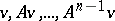form a basis for, i.e. such that the pairis completely reachable (see also Pole assignment problem; Majorization ordering; System of subvarieties; Frobenius matrix).

A vectorin an (infinite-dimensional) Banach space or Hilbert space with an operatoron it is said to be cyclic if the linear combinations of the vectors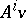,, form a dense subspace, [a1].

More generally, letbe a subalgebra of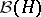, the algebra of bounded operators on a Hilbert space. Then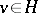is cyclic ifis dense in, [a2], [a5].

Ifis a unitary representation of a (locally compact) groupin, thenis called cyclic if the linear combinations of the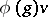,, form a dense set, [a3], [a4]. For the connection between positive-definite functions onand the cyclic representations (i.e., representations that admit a cyclic vector), see Positive-definite function on a group. An irreducible representation is cyclic with respect to every non-zero vector.

How to Cite This Entry:
Cyclic vector. Encyclopedia of Mathematics. URL: http://encyclopediaofmath.org/index.php?title=Cyclic_vector&oldid=18561
This article was adapted from an original article by M. Hazewinkel (originator), which appeared in Encyclopedia of Mathematics - ISBN 1402006098. See original article